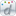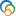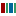The Library of Congress > Linked Data Service > LC Subject Headings (LCSH)

# Pythagorean theorem

• ### Variants

• Pythagoras' theorem
• Pythagorean proposition
• Theorem, Pythagorean

• ### Closely Matching Concepts from Other Schemes

•Pythagore, Théorème de•Pythagorean theorem•Pythagorean theoremLabel from public data source Wikidata
• ### Earlier Established Forms

• Pythagorean proposition
• ### Sources

• found: McGraw-Hill dict. sci. tech.(Pythagoream theorem: This theorem states that in any right triangle the square on the hypotenuse is equal to the sum of the squares on the other two sides. More than 100 different proofs have been given for this extremely important theorem of euclidean plane geometry, which is named for the Greek philosopher and mathematician Pythagoras (6th cent. B.C.))
• found: World Book(Pythagoras' theorem)
• found: Web. 3(Pythagorean theorem)

• QA460.P8
• ### Change Notes

• 2000-05-17: new
• 2000-06-16: revised

# Suggest terminology

The LC Linked Data Service welcomes any suggestions you might have about terminology used for a given heading or concept.

Would you like to suggest a change to this heading?

Please provide your name, email, and your suggestion so that we can begin assessing any terminology changes.

Fields denoted with an asterisk (*) are required.